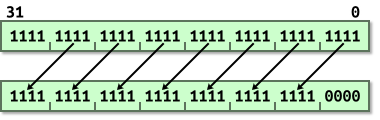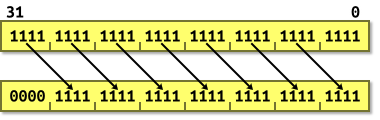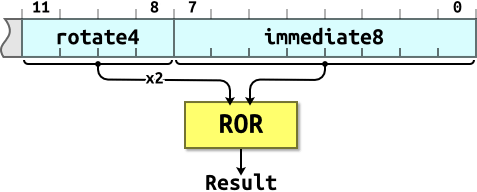# ARM: Introduction to ARMhttp://www.manostaxx.com

## Why Learn Assembly Language?

• Ideally we would never need to write assembly language.
• Because it requires more effort to code than HLLs like C.
• But C is an abstraction: The code that you write is not what the CPU really executes.
• Sometimes compilers aren’t very good.
• They may produce unnecessary extra code which we call slop.
• Slop increases size and decreases speed.
• To dispense with the slop we need to instruct the machine in its native language.

## Not a Trivial Mapping

There are features available to the assembly language programmer which don’t map onto C:

• Processor flags
• the carry flag.
• Vector operations
• SIMD instructions.
• Bulk transfers
• Specialised operations
• population count.
• Atomic test-and-set instructions
• mutexes.
• Other on-chip hardware
• MAC units.

The benefits of these features may be lost if plain, or naïve, C code is used.

## Instruction Sets

Modern ARM processors have several instruction sets:

• The fully-featured 32-bit ARM instruction set,
• The more restricted, but space efficient, 16-bit Thumb instruction set,
• The newer mixed 16/32-bit Thumb-2 instruction set,
• Jazelle DBX for Java byte codes,
• The NEON 64/128-bit SIMD instruction set,
• The VFP vector floating point instruction set.

For the purposes of this course we are only interested in the ARM and Thumb instruction sets.

## Registers

ARM has sixteen registers visible at any one time. They are named R0 to R15. All are 32 bits wide.The registers may also be referred to by the following aliases:All of the registers are general purpose, save for:

• R13 / SP
• which holds the stack pointer.
• R14 / LR
• R15 / PC
• which holds the program counter.

In addition to the main registers there is also a status register:CPSR is the current program status register. This holds flags: results of arithmetic and logical operations.

## Program Counter

• When in ARM mode:
• Instructions are 32 bits wide.
• All instructions must be word-aligned.
• The PC is in bits [31:2] and bits [1:0] are undefined.
• When in Thumb mode:
• Instructions are 16 bits wide.
• All instructions must be halfword-aligned.
• The PC is in bits [31:1] and bit 0 is undefined.

## Instruction Syntax

`<operation>{cond}{flags} Rd,Rn,Operand2`
• `<operation>`
• A three-letter mnemonic, e.g. `MOV` or `ADD`.
• `{cond}`
• An optional two-letter condition code, e.g. `EQ` or `CS`.
• `{flags}`
• An optional additional flags. e.g. `S`.
• `Rd`
• The destination register.
• `Rn`
• The first source register.
• `Operand2`
• A flexible second operand.

## Organisation

• `Rd` — destination register
• `Rn` — source register
• `Rm` — source register

e.g. `ADD R0,R1,R2`.
`Rn` is used directly but `Rm` is passed through the barrel shifter; a functional unit which can rotate and shift values. The result of this is called Operand2.The two operands are processed by the ALU and the result written to `Rd`.

## Movement

`<operation>{cond}{S} Rd,Operand2`

`<operation>`

• `MOV` – move
• Rd := Operand2
• `MVN` – move NOT
• Rd := 0xFFFFFFFF EOR Operand2

## Examples

• `MOV r0, #42`
• Move the constant 42 into register R0.
• `MOV r2, r3`
• Move the contents of register R3 into register R2.
• `MVN r1, r0`
• R1 = NOT(R0) = -43
• `MOV r0, r0`
• A NOP (no operation) instruction.

## Arithmetic Instructions

`<operation>{cond}{S} Rd,Rn,Operand2`

`<operation>`

• `ADD` – Add
• Rd := Rn + Operand2
• `ADC` – Add with Carry
• Rd := Rn + Operand2 + Carry
• `SUB` – Subtract
• Rd := Rn − Operand2
• `SBC` – Subtract with Carry
• Rd := Rn − Operand2 − NOT(Carry)
• `RSB` – Reverse Subtract
• Rd := Operand2 − Rn
• `RSC` – Reverse Subtract with Carry
• Rd := Operand2 − Rn − NOT(Carry)

## Examples

• `ADD r0, r1, r2`
• R0 = R1 + R2
• `SUB r5, r3, #10`
• R5 = R3 − 10
• `RSB r2, r5, #0xFF00`
• R2 = 0xFF00 − R5

## Logical Instructions

`<operation>{cond}{S} Rd,Rn,Operand2`

`<operation>`

• `AND` – logical AND
• Rd := Rn AND Operand2
• `EOR` – Exclusive OR
• Rd := Rn EOR Operand2
• `ORR` – logical OR
• Rd := Rn OR Operand2
• `BIC` – Bitwise Clear
• Rd := Rn AND NOT Operand2

## Examples

• `AND r8, r7, r2`
• R8 = R7 & R2
• `ORR r11, r11, #1`
• R11 |= 1
• `BIC r11, r11, #1`
• R11 &= ~1
• `EOR r11, r11, #1`
• R11 ^= 1

## Compare Instructions

`<operation>{cond} Rn,Operand2`

`<operation>`

• `CMP` – compare
• Flags set to result of (Rn − Operand2).
• `CMN` – compare negative
• Flags set to result of (Rn + Operand2).
• `TST` – bitwise test
• Flags set to result of (Rn AND Operand2).
• `TEQ` – test equivalence
• Flags set to result of (Rn EOR Operand2).

Comparisons produce no results – they just set condition codes. Ordinary instructions will also set condition codes if the “S” bit is set. The “S” bit is implied for comparison instructions.

## Examples

• `CMP r0, #42`
• Compare R0 to 42.
• `CMN r2, #42`
• Compare R2 to -42.
• `TST r11, #1`
• Test bit zero.
• `TEQ r8, r9`
• Test R8 equals R9.
• `SUBS r1, r0, #42`
• Compare R0 to 42, with result.

## Barrel Shifter

The barrel shifter is a functional unit which can be used in a number of different circumstances. It provides five types of shifts and rotates which can be applied to Operand2. (These are not operations themselves in ARM mode.)

## `LSL` – Logical Shift Left

Example: Logical Shift Left by 4.Equivalent to `<<` in C.

## `LSR` – Logical Shift Right

Example: Logical Shift Right by 4.Equivalent to `>>` in C. i.e. unsigned division by a power of 2.

## `ASR` – Arithmetic Shift Right

Example: Arithmetic Shift Right by 4, positive value.Example: Arithmetic Shift Right by 4, negative value.Equivalent to `>>` in C. i.e. signed division by a power of 2.

## `ROR` – Rotate Right

Example: Rotate Right by 4.Bit rotate with wrap-around.

## `RRX` – Rotate Right Extended

Example: Rotate Right Extended.33-bit rotate with wrap-around through carry bit.

## Examples

• `MOV r0, r0, LSL #1`
• Multiply R0 by two.
• `MOV r1, r1, LSR #2`
• Divide R1 by four (unsigned).
• `MOV r2, r2, ASR #2`
• Divide R2 by four (signed).
• `MOV r3, r3, ROR #16`
• Swap the top and bottom halves of R3.
• `ADD r4, r4, r4, LSL #4`
• Multiply R4 by 17. (N = N + N * 16)
• `RSB r5, r5, r5, LSL #5`
• Multiply R5 by 31. (N = N * 32 – N)

## Operand2

Operand2 is the flexible second operand to most instructions. It can take one of three different forms:

• Immediate value.
• An 8-bit number rotated right by an even number of places.
• Register shifted by value.
• A 5-bit unsigned integer shift.
• Register shifted by register.
• The bottom 8 bits of a register.

## Examples

• Immediate values:
• `MOV r0, #42`
• `ORR r1, r1, #0xFF00`
• Registers shifted by values:
• `MOV r2, r2, LSR #1`
• `RSB r10, r5, r14, ASR #14`
• Registers shifted by registers:
• `BIC r11, r11, r1, LSL r0`
• `CMP r9, r8, ROR r0`

## Immediate Values

• You can’t fit an arbitrary 32-bit value into a 32-bit instruction word.
• ARM data processing instructions have 12 bits of space for values in their instruction word. This is arranged as a four-bit rotate value and an eight-bit immediate value:The 4-bit rotate value stored in bits 11-8 is multiplied by two giving a range of 0-30 in steps of two.
Using this scheme we can express immediate constants such as:

• `0x000000FF`
• `0x00000FF0`
• `0xFF000000`
• `0xF000000F`

But immediate constants such as:

• `0x000001FE`
• `0xF000F000`
• `0x55550000`

…are not possible.
An assembler will convert big values to the rotated form. Impossible values will cause an error.
Some assemblers will use other tricks such as using `MVN` instead of `MOV` to form the bitwise complement of the required constant. For example the impossible instruction `MOV r0,#0xFFFFFFFF` could be assembled as `MVN r0,#0`.

## Remarks

The impact of this is that some constants are “ARM friendly”. Some are not. Study of the numbers you’re using can sometimes reveal scope for optimisation.

You can form constants wider than those available in a single instruction by using a sequence of instructions to build up the constant. For example:

```MOV r2, #0x55           ; R2 = 0x00000055
ORR r2, r2, r2, LSL #8  ; R2 = 0x00005555
ORR r2, r2, r2, LSL #16 ; R2 = 0x55555555
```

…or load the value from memory:

```LDR r2, =0x55555555
```

The pseudo-instruction `LDR Rx,=const` tries to form the constant in a single instruction, if possible, otherwise it will generate an `LDR`.

## Branch Instructions

So how do we implement control structures like `for` and `while` loops? Branch instructions are used to alter control flow.

`<operation>{cond} <address>`
• `B` – Branch
• `BL` – Branch with Link

How do we return from the subroutine which `BL` invoked?
`MOV pc, r14`
or
`BX r14` (on ARMv4T or later)

## Examples

Branching forward, to skip over some code:

```    ...            ; some code here
...            ; more code here
fwd
```

Branching backwards, creating a loop:

```back
...            ; more code here
```

Using `BL` to call a subroutine:

```    ...
...
BL  calc       ; call 'calc'
...            ; returns to here
...
calc               ; function body
ADD r0, r1, r2 ; do some work here
MOV pc, r14    ; PC = R14 to return
```

## Remarks

Branches are PC-relative. +/-32M range (24 bits × 4 bytes).
Since ARM’s branch instructions are PC-relative the code produced is position independent — it can execute from any address in memory. Certain systems such as BREW use this.
How can we perform longer branches which access the full 32-bit address space?
You can set up the LR manually if needed, then load into PC:

```MOV lr,pc
LDR pc,=dest```

Continue at: http://www.davespace.co.uk/arm/introduction-to-arm/branch.html
The text above is owned by the site above referred.